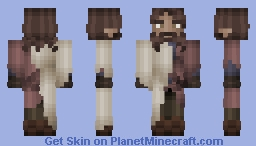# Mahrus 2• 8
• 1
~=~=~=~=~=~=~=~=~=~=~=~=~=~=~=~=~=~=~=~=~=~=~=~=~=~=~=~=~=~=~=~=~=~=~=~=~=~=~=~=~=~=~=~=~=~

Character Name: Mahrus Fanayae
Race: Desert Elf
Hair: Dark Brown
Eyes: Hazel

This skin is used on The Book of Ventura by YeYePapu

~=~=~=~=~=~=~=~=~=~=~=~=~=~=~=~=~=~=~=~=~=~=~=~=~=~=~=~=~=~=~=~=~=~=~=~=~=~=~=~=~=~=~=~=~=~